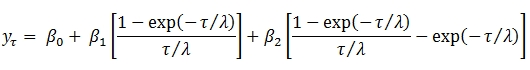# SQL Server Nelson Siegel coefficients for a zero coupon curve

NSCOEF

Updated: 29 Mar 2013

Use the table-valued function NSCOEF to calculate the Nelson Siegel coefficients for a zero coupon curve. Nelson and Siegel suggested calculating the yield curve at a point using this formula:To find the coefficients, the program uses ordinary least squares to calculate the values of B0, B1, and B2 for any value of λ and simply finds the value of λ (to 4 decimal places) which has the smallest r2. There may be more than solution; however the function only returns the first one that it finds.
Syntax
SELECT * FROM [wctFinancial].[wct].[NSCOEF](
<@YieldCurve_RangeQuery, nvarchar(max),>)
Arguments
@YieldCurve_RangeQuery
a T-SQL statement, as a string, that specifies the maturities (as measured in years) and their zero coupon rates to be used as in calculating the Nelson Siegel coefficients.
Return Type
RETURNS TABLE (
[B0] [float] NULL,
[B1] [float] NULL,
[B2] [float] NULL,
[Tau] [float] NULL,
[RMSE] [float] NULL
)

 Column Column Description B0 The first coefficient B1 The second coefficient B2 The third coefficient Tau Tau RMSE Residual sum of squares. SQUARE(SUM(y – y))

Remarks
·         The function is insensitive to order; it does not matter what order the dates and rates are passed in.
·         See NELSONSIEGEL to calculate the interpolated values using the Nelson Siegel coefficients.
·         See NSCOEF2 for another way to calculate the Nelson Siegel coefficients.
Examples
SELECT *
FROM wct.NSCOEF(
'SELECT 1,0.0028 UNION ALL
SELECT 2,0.0056 UNION ALL
SELECT 3,0.0085 UNION ALL
SELECT 5,0.0164 UNION ALL
SELECT 7,0.0235 UNION ALL
SELECT 10,0.0299 UNION ALL
SELECT 20,0.0382 UNION ALL
SELECT 30,0.0406') k
This produces the following result.
B0                  B1                  B2    Tau                   RMSE
------------------ ------------------- ------------------- ------ ----------------------
0.0466701067626488 -0.0409398575156674 -0.0635075128703469 1.6545    9.8912932859058E-07

This example uses the LINEST_q function to demonstrate the calculation of the coefficients when tau is equal to 1.6545.

DECLARE @tau as float = 1.6545

SELECT *
FROM wct.LINEST_q(
REPLACE('SELECT zr
,(1 - EXP(-cast(mat as float) / @tau)) / (cast(mat as float) / @tau) as x1
,(1 - EXP(-cast(mat as float) / @tau)) / (cast(mat as float) / @tau) - EXP(-cast(mat as float) / @tau) as x2
FROM (
SELECT 1,0.0028 UNION ALL
SELECT 2,0.0056 UNION ALL
SELECT 3,0.0085 UNION ALL
SELECT 5,0.0164 UNION ALL
SELECT 7,0.0235 UNION ALL
SELECT 10,0.0299 UNION ALL
SELECT 20,0.0382 UNION ALL
SELECT 30,0.0406
)n(mat,zr)','@tau',CAST(@tau as varchar(max)))
,1
,'True')
WHERE stat_name in('m','ss_resid')

This produces the following result.
stat_name          idx               stat_val
---------- ----------- ----------------------
m                    0     0.0466701067626488
m                    1    -0.0409398575156674
m                    2    -0.0635075128703469
ss_resid          NULL    9.8912932859058E-07

### SupportCopyright 2008-2021 Westclintech LLC         Privacy Policy        Terms of Service